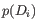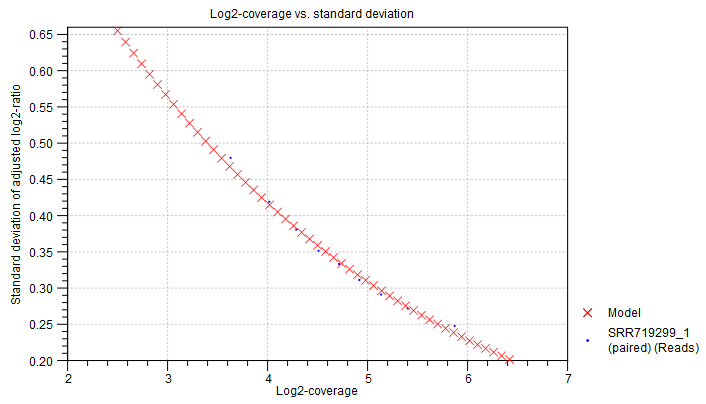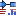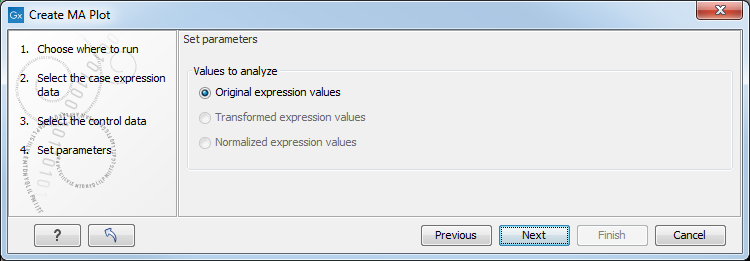## MA plot

The MA plot is a scatter rotated by. For two samples of expression values it plots for each gene the difference in expression against the mean expression level. MA plots are often used for quality control, in particular, to assess whether normalization and/or transformation is required.

You can create an MA plot comparing two samples:

Toolbox | Microarray Analysis ()| General Plots () | Create MA Plot ()

In the first two dialogs, select two samples ( (), () or ()): the first must be the case expression data, and the second the control data. Clicking Next will display a dialog as shown in figure 31.60.Figure 31.60: Selecting which values the MA plot should be based on.

In this dialog, you select the values to be used for creating the MA plot (see Selecting transformed and normalized values for analysis).

Click Finish to start the tool.

Subsections